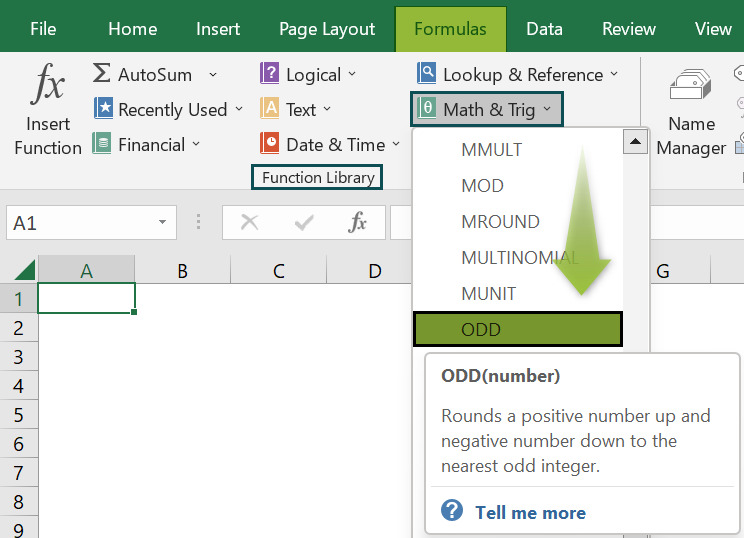# ODD Function In ExcelArticle byExcelMojo Team## What Is ODD Function In Excel?

The ODD Function in Excel rounds the numeric values in a dataset to its nearest odd number away from zero. On a number scale, it rounds up toward the right for a positive integer input and toward the left for a negative integer input.

The ODD Excel function is an inbuilt Math & Trig function, so we can insert the formula from the “Function Library” or enter it directly in the worksheet.

For example, the image below depicts the Values in column A. We will apply the ODD Excel Function to find the absolute values as the output.

Select cell B2, enter the formula =ODD(A2), and press the “Enter” key.

The result is ‘3’, as shown above.

###### Key Takeaways
• The ODD Function in Excel finds the nearest odd number of any numeric value or the results of formulas like SUM, AVERAGE, etc.
• Every positive and negative numeric value is rounded up to the nearest odd integer away from zero, as on the number scale.
• In the case of a decimal number cell value, the formula ignores the numbers after the decimal point, considers only the whole number, and rounds it up to the nearest odd number.
• If the number is an odd integer, the number itself is the result.

### ODD() Excel Formula

The syntax of the ODD Excel Formula is,

The argument of the ODD Excel Formula is,

• number: It is a mandatory argument. The number is to be rounded up to an odd integer.

### How To Use ODD Excel Function?

We can use the ODD Excel function in 2 ways, namely,

1. Access from the Excel ribbon.
2. Enter in the worksheet manually.

#### Method #1 – Access from the Excel ribbon

Choose an empty cell that will contain the result → select the “Formulas” tab → go to the “Function Library” group → click the “Math & Trig” option drop-down → select the “ODD” function, as shown below.

The “Function Arguments” window appears. Enter the arguments in the “Number” field, and click “OK”, as shown below.

#### Method #2 – Enter in the worksheet manually

1. Select an empty cell for the output.
2. Type =ODD( in the selected cell. [Alternatively, type =O and double-click and then the ODD function from the list of suggestions shown by Excel.]
3. Enter the arguments as cell values or cell references and close the brackets.
4. Press the “Enter” key.

The succeeding example depicts the values, and we will find the odd value of the given values using the ODD Excel Function.

In the table, the data is,

• Column A contains the Value.
• Column B contains the Output.

The steps to find the value using the ODD exce; formula are,

1. Select cell B2, and =ODD(A2), i.e., ‘number’ as A2.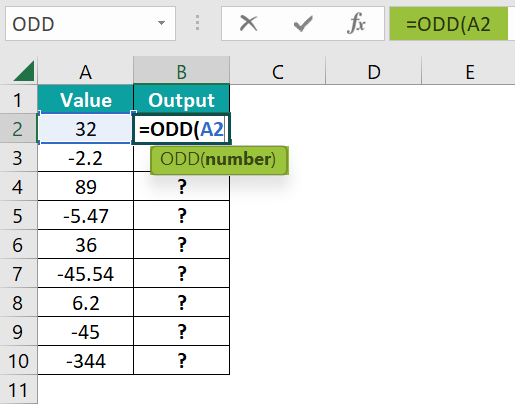2. Press the “Enter” key. The result is “33”, as shown below.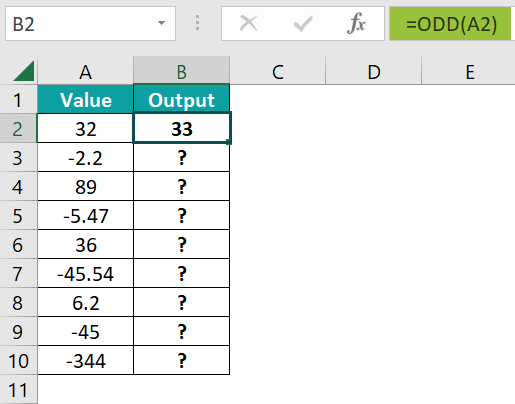3. Drag the formula from cell B2 to B10 using the fill handle. The output is shown below.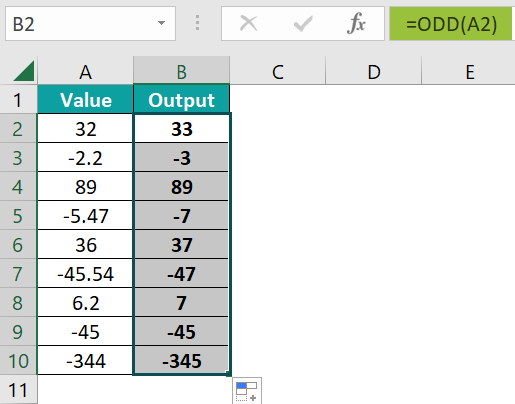### Examples

We will consider some advanced scenarios using the ODD function in Excel examples.

#### Example #1

The succeeding example depicts the values, and we will find the odd value of the given values using the ODD Excel Function.

In the table, the data is,

• Column A contains the Description of the formula.
• Column B contains the Output.

The procedure to find the nearest odd value using the ODD formula is,

Enter the formulas as follows,

• =ODD(10) in cell B3.
• =ODD(-10) in cell B4.
• =ODD(A1) in cell B5.
• Press the “Enter” key every time we enter the formula.

The results in cells B3 to B5 are “11, -11, & -21”, as shown above.

#### Example #2

The succeeding example depicts two sets of values. Using the ODD Excel Function, we will subtract the numbers, and then round the subtracted values to the nearest odd value.

In the table, the data is,

• Column A contains Value 1.
• Column B contains Value 2.
• Column C will display the Subtracted values.
• Column D contains the ODD Result.

The steps to perform the required calculations are,

• Step 1: Select cell C2, enter the formula =A2-B2, and press “Enter”. The result is 13, as shown below.
• Step 2: Drag the formula from cell C2 to C5 using the excel fill handle. Now, we have the subtracted values, as shown below.
• Step 3: Next, select cell D2, enter the formula =ODD(C2), and press the “Enter” key. The result is “13”, as shown below.
• Step 4: Drag the formula from cell D2 to D5 using the fill handle. The output is shown below.

#### Example #3

The succeeding example depicts the values, and we will calculate the sum of the given values, and then find the nearest odd value of the sum result using the ODD Excel Function.

In the table, the data is

• Column A contains the Value.
• Column B contains the Actual Result.
• Column C contains the ODD Result.

The steps to calculate the value by the ODD Excel Function are as follows:

• Step 1: Select cell B2, enter the formula =SUM(A2:A6), and press “Enter”. The result is “-2028”, as shown below. Cell B3 is for our reference.
• Step 2: Select cell C2, enter the formula =ODD(B2), and press the “Enter” key. The result is “-2029”, as shown below. Cell C3 is for our reference.

[Special Note: Alternatively, we can use the direct formula as =ODD(SUM(A2:A6)) in the target cell C2. We will get the same output, as shown below. Cell C3 is for our reference.]

### Important Things To Note

• The function considers only numbers as input. We will get the “#VALUE!” error if the input is non-numeric or even alphanumeric.
• If the cell value is blank or empty, by default, the output is 1.
• We get the “#NAME?” error when the function’s name or formula is incorrectly entered, or if the cell value is non-numeric, without double-quotes.

1. What does the ODD function do in Excel?

The ODD Function in Excel finds and returns the odd numbers in a series of numbers.

The syntax of the ODD function is =ODD(number)

2. How does the ODD function work?

The Excel ODD Function works as shown below.
1) Select an empty cell for the output.
2) Type =ODD( in the selected cell. [Alternatively, type =O and double-click and then the ODD function from the list of suggestions shown by Excel.]
3) Enter the arguments as cell values or cell references and close the brackets.
4) Press the “Enter” key.
For example, using the ODD Excel Function, we will find the odd value for the average of the given values.

In the table, the data is,
Column A contains the Value.
Column B contains the ODD Result.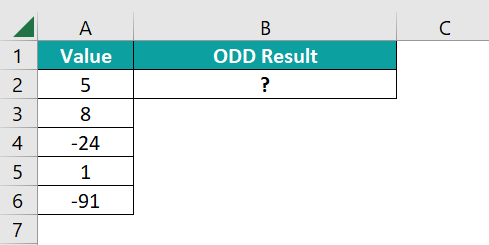The procedure to find the value by the ODD formula is,

Select cell B2, enter the formula =ODD(AVERAGE(A2:A6), and press the “Enter” key.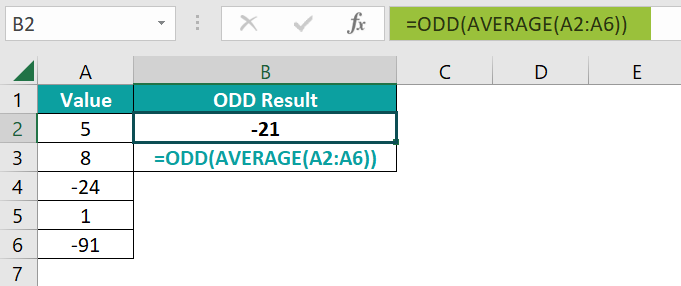The result is “-21”, as shown above.

3. Where is the ODD Function in Excel?

The ODD Function in Excel is found and inserted as follows:

Choose an empty cell → select the “Formulas” tab → go to the “Function Library” group → click the “Math & Trig” option drop-down → select the “ODD” function, as shown below.